# RD Sharma Class 8 Solutions Chapter 3 Squares and Square Roots Ex 3.1

In this chapter, we provide RD Sharma Class 8 Solutions Chapter 3 Squares and Square Roots Ex 3.1 for English medium students, Which will very helpful for every student in their exams. Students can download the latest RD Sharma Class 8 Solutions Chapter 3 Squares and Square Roots Ex 3.1 Maths pdf, free RD Sharma Class 8 Solutions Chapter 3 Squares and Square Roots Ex 3.1 Maths book pdf download. Now you will get step by step solution to each question.

### RD Sharma Solutions for Class 8 Chapter 3 Squares and Square Roots Ex 3.1 Download PDF

Question 1.
Which of the following numbers are perfect squares ?
(i)484
(ii) 625
(iii) 576
(iv) 941
(v) 961
(vi) 2500
Solution: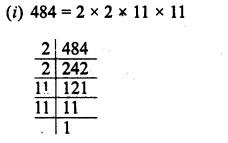Grouping the factors in pairs, we have left no factor unpaired
∴ 484 is a perfect square of 22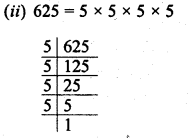∴ Grouping the factors in pairs, we have left no factor unpaired
∴ 625 is a perfect square of 25.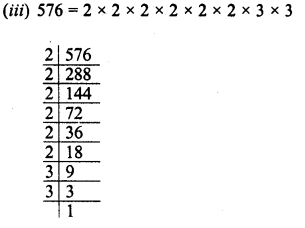Grouping the factors in pairs, we see that no factor is left unpaired
∴ 576 is a perfect square of 24
(iv) 941 has no prime factors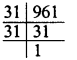∴ 941 is not a perfect square.
(v) 961 =31 x 31
Grouping the factors in pairs, we see that no factor is left unpaired
∴ 961 is a perfect square of 31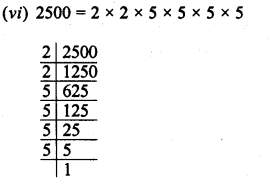Grouping the factors in pairs, we see that no factor is left impaired
∴ 2500 is a perfect square of 50 .

Question 2.
Show that each of the following* numbers is a perfect square. Also find the number whose square is the given number in each case :
(i) 1156
(ii) 2025
(iii) 14641
(iv) 4761
Solution: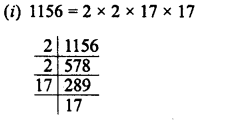Grouping the factors in pairs, we see that no factor is left unpaired
∴ 1156 is a perfect square of 2 x 17 = 34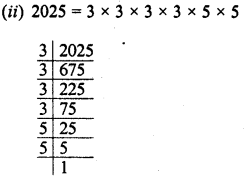Grouping the factors in pairs, we see that no factor is left unpaired
2025 is a perfect square of 3 x 3 x 5 =45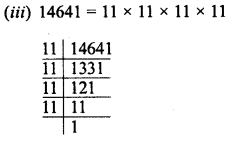Grouping the factors in pairs, we see that no factor is left unpaired
∴ 14641 is a perfect square of 11×11 = 121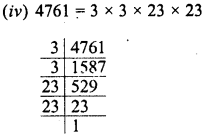Grouping the factors in pairs, we see that no factor is left unpaired
∴ 4761 is a perfect square of 3 x 23 = 69

Question 3.
Find the smallest number by which the given number must be multiplied so that the product is a perfect square.
(i) 23805
(ii) 12150
(iii) 7688
Solution: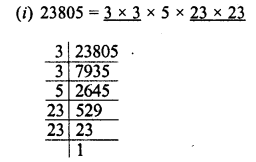Grouping the factors in pairs of equal factors, we see that 5 is left unpaird
∴ In order to complete the pairs, we have to multiply 23805 by 5, then the product will be the perfect square.
Requid smallest number = 5
(ii) 12150 = 2 x 3 x 3×3 x 3×3 x 5×5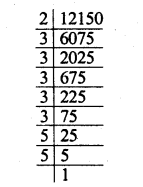Grouping the factors in pairs of equal factors, we see that factors 2 and 3 are left unpaired
∴ In order to complete the pairs, we have to multiply 12150 by 2 x 3 =6 i.e., then the product will be the complete square.
∴ Required smallest number = 6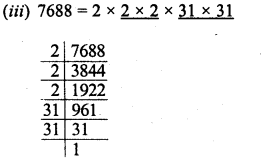Grouping the factors in pairs of equal factors, we see that factor 2 is left unpaired
∴ In order to complete the pairs we have to multiply 7688 by 2, then the product will be the complete square
∴ Required smallest number = 2

Question 4.
Find the smallest number by which the given number must be divided so that the resulting number is a perfect square.
(i) 14283
(ii) 1800
(iii) 2904
Solution: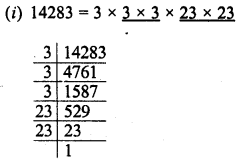Grouping the factors in pairs of equal factors, we see that factors we see that 3 is left unpaired
Deviding by 3, the quotient will the perfect square.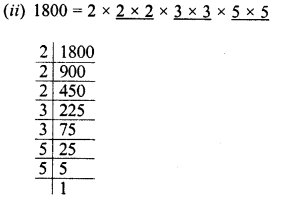Grouping the factors in pair of equal factors, we see that 2 is left unpaired.
∴ Dividing by 2, the quotient will be the perfect square.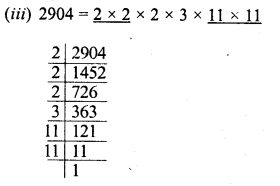Grouping the factors in pairs of equal factors, we see that 2 x 3 we left unpaired
∴ Dividing by 2 x 3 = 6, the quotient will be the perfect square.

Question 5.
Which of the following numbers are perfect squares ?
11, 12, 16, 32, 36, 50, 64, 79, 81, 111, 121
Solution:
11 is not a perfect square as 11 = 1 x 11
12 is not a perfect square as 12 = 2×2 x 3
16 is a perfect square as 16 = 2×2 x 2×2
32 is not a perfect square as 32 = 2×2 x 2×2 x 2
36 is a perfect square as 36 = 2×2 x 3×3
50 is not a perfect square as 50 = 2 x 5×5
64 is a perfect square as 64 = 2×2 x 2×2 x 2×2
79 is not a perfect square as 79 = 1 x 79
81 is a perfect square as 81 = 3×3 x 3×3
111 is not a perfect square as 111 = 3 x 37
121 is a perfect square as 121 = 11 x 11
Hence 16, 36, 64, 81 and 121 are perfect squares.

Question 6.
Using prime factorization method, find which of the following numbers are perfect squares ?
∴ 189,225,2048,343,441,2916,11025,3549
Solution: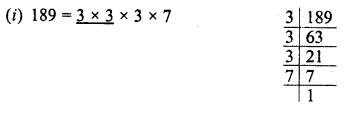Grouping the factors in pairs, we see that are 3 and 7 are left unpaired
∴ 189 is not a perfect square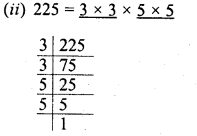Grouping the factors in pairs, we see no factor left unpaired
∴ 225 is a perfect square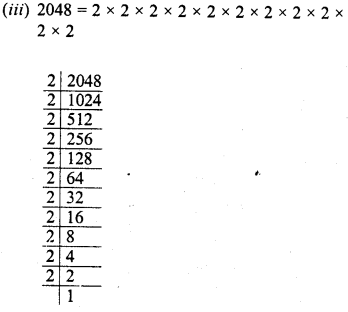Grouping the factors in pairs, we see no factor left unpaired
∴ 2048 is a perfect square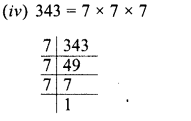Grouping the factors in pairs, we see that one 7 is left unpaired
∴ 343 is not a perfect square.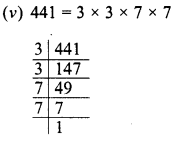Grouping the factors in pairs, we see that no factor is left unpaired
∴ 441 is a perfect square.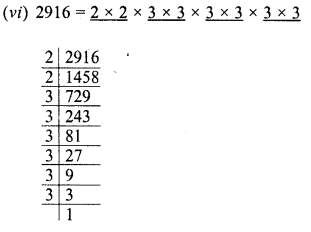Grouping the factors in pairs, we see that no factor is left unpaired
∴ 2916 is a perfect square.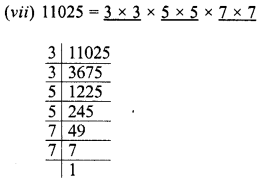Grouping the factors in pairs, we see that no factor is left unpaired
∴ 11025 is a perfect square.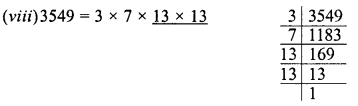Grouping the factors in pairs, we see that 3, no factor 7 are left unpaired
∴ 3549 is a perfect square

Question 7.
By what number should each of the following numbers be multiplied to get a perfect square in each case ? Also, find the number whose square is the new number.
(i) 8820
(ii) 3675
(iii) 605
(iv) 2880
(v) 4056
(vi) 3468
Solution: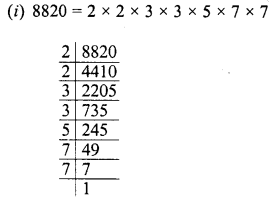Grouping the factors in pairs, we see that 5 is left unpaired
∴ By multiplying 8820 by 5, we get the perfect square and square root of product will be
= 2 x 3 x 5 x 7 = 210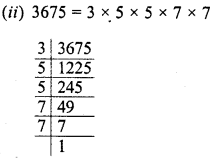Grouping the factors in pairs, we see that 3 is left unpaired
∴ Multiplying 3675 by 3, we get a perfect square and square of the product will be
= 3 x 5 x 7 = 105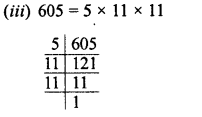Grouping the factors in pairs, we see that 5 is left unpaired
∴ Multiplying 605 by 5, we get a perfect square and square root of the product will be
= 5 x 11 =55Grouping the factors in pairs, we see that 5 is left unpaired
∴ Multiplying 2880 by 5, we get the perfect square.
Square rooi of product will be = 2 x 2 * 2 – 3 x 5 = 120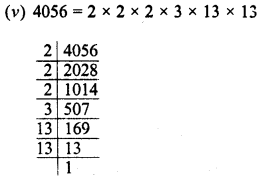Grouping the factors in pairs, we see that 2 and 3 are left unpaired
∴ Multiplying 4056 by 2 x 3 i.e., 6, we get the perfect square.
and square root of the product will be
= 2 x 2 x 3 x 13 = 156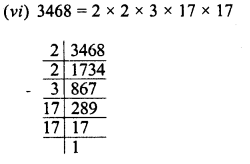Grouping the factors in pairs, we see that 3 is left unpaired
∴ Multiplying 3468 by 3 we get a perfect square, and square root of the product will be 2 x 3 x 17 = 102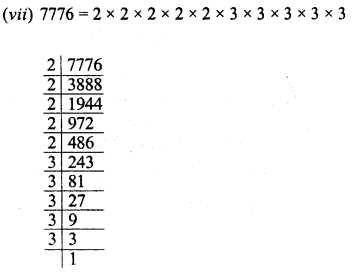Grouping the factors in pairs, we see that 2 and 3 are left unpaired
∴ Multiplying 7776 by 2 x 3 or 6 We get a perfect square and square root of the product will be
= 2 x 2 x 2 x 3 x 3 x 3 = 216

Question 8.
By what numbers should each of the following be .divided to get a perfect square in each case ? Also find the number whose square is the new number.
(i) 16562
(ii) 3698
(iii) 5103
(iv) 3174
(v) 1575
Solution: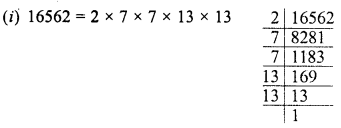Grouping the factors in pairs, we see that 2 is left unpaired
∴ Dividing by 2, we get the perfect square and square root of the quotient will be 7 x 13 = 91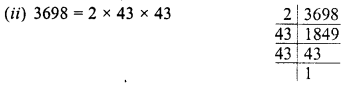Grouping the factors in pairs, we see that 2 is left unpaired,
∴ Dividing 3698 by 2, the quotient is a perfect square
and square of quotient will be = 43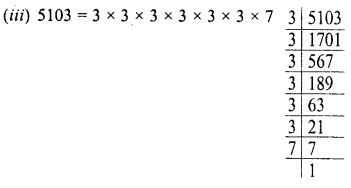Grouping the factors in pairs, we see that 7 is left unpaired
∴ Dividing 5103 by 7, we get the quotient a perfect square.
and square root of the quotient will be 3 x 3 x 3 = 27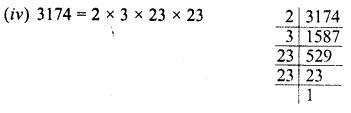Grouping the factors iq pairs, we see that 2 and 3 are left unpaired
∴ Dividing 3174 by 2 x 3 i.e. 6, the quotient will be a perfect square and square root of the quotient will be = 23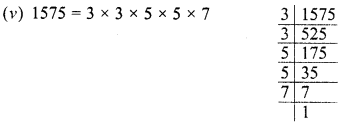Grouping the factors in pairs, we find that 7 is left unpaired i
∴ Dividing 1575 by 7, the quotient is a perfect square
and square root of the quotient will be = 3 x 5 = 15

Question 9.
Find the greatest number of two digits which is a perfect square.
Solution:
The greatest two digit number = 99 We know, 92 = 81 and 102 = 100 But 99 is in between 81 and 100
∴ 81 is the greatest two digit number which is a perfect square.

Question 10.
Find the least number of three digits which is perfect square.
Solution:
The smallest three digit number =100
We know that 92 = 81, 102 = 100, ll2 = 121
We see that 100 is the least three digit number which is a perfect square.

Question 11.
Find the smallest number by which 4851 must be multiplied so that the product becomes a perfect square.
Solution:
By factorization: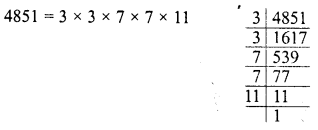Grouping the factors in pairs, we see that 11 is left unpaired
∴ The least number is 11 by which multiplying 4851, we get a perfect square.

Question 12.
Find the smallest number by which 28812 must be divided so that the quotient becomes a perfect square.
Solution:
By factorization,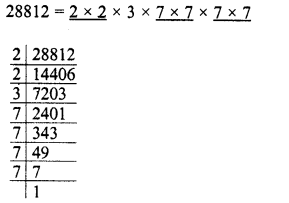Grouping the factors in pairs, we see that 13 is left unpaired
∴ Dividing 28812 by 3, the quotient will be a perfect square.

Question 13.
Find the smallest number by which 1152 must be divided so that it becomes a perfect square. Also find the number whose square is the resulting number.
Solution:
By factorization,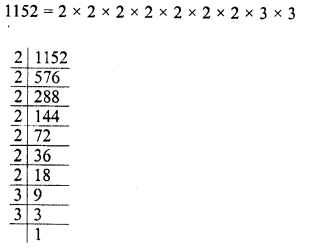All Chapter RD Sharma Solutions For Class 8 Maths

—————————————————————————–

All Subject NCERT Exemplar Problems Solutions For Class 8

All Subject NCERT Solutions For Class 8

*************************************************

I think you got complete solutions for this chapter. If You have any queries regarding this chapter, please comment on the below section our subject teacher will answer you. We tried our best to give complete solutions so you got good marks in your exam.

If these solutions have helped you, you can also share rdsharmasolutions.in to your friends.